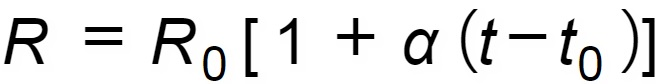# Wire Resistance

## Calculator

Type wire material, temperature and cross-sectional area:

Temperature coefficient: (ºC -1)
Resistivity at 20ºC: (Ω mm2/m)

Wire area: (mm2)

Temperature: (ºC)

Results at ºC::
Resistivity: (Ω mm2/m)
Resistance per meter: (Ω / m)

## NOTES

### Introduction:

Resistance depends on temperature. Sometimes, we need to calculate the correct value for a given temperature as a change of resistance will lead to a change in the voltage drop of the circuits. In case of big temperature changes voltage drop variation can be relevant. In general, the higher the temperature the lower the conductivity and the higher the resistance. This calculator can be used for wires of copper or aluminum. It can also be used for other materials as long as temperature coefficient and resistivity at 20ºC temperature are known.
It is important to advice that not all materials have a linear behavior regarding changes in resistivity vs temperature. In these cases, please do not use this calculator.

### Equation:R: resistance at t temperature
Ro: resistance at reference temperature (20ºC)
t: temperature for the calculation
to: 20ºC

### Skin and proximity effects:

For alternating currents, the value of the resistance depends also on skin and proximity effects that are relevant for wires with a big cross-sectional area. This calculator does not consider these effects. They can be calculated following the standard UNE21144. However, these effects are usually considered neglibible for many low voltage installations.